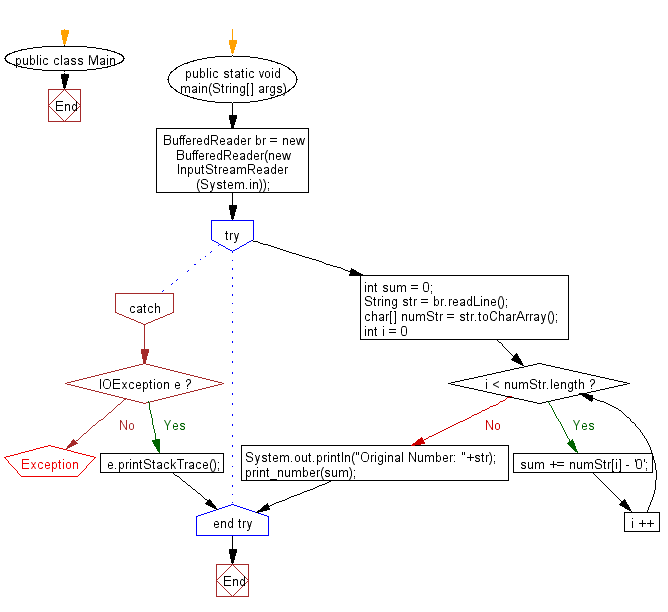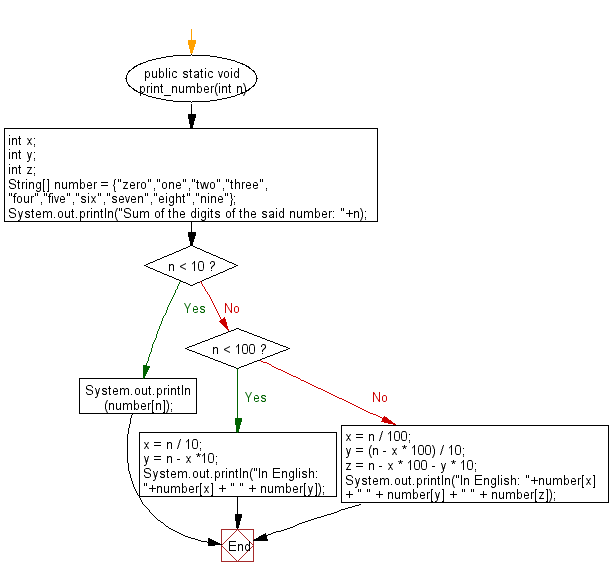﻿ Java exercises: Program than read an integer and calculate the sum of its digits and write the number of each digit of the sum in English - w3resource# Java Exercises: Program than read an integer and calculate the sum of its digits and write the number of each digit of the sum in English

## Java Basic: Exercise-87 with Solution

Write a Java program than read an integer and calculate the sum of its digits and write the number of each digit of the sum in English.

Sample Solution:

Java Code:

``````import java.io.*;

public class Main {
public static void main(String[] args) {

try {
int sum = 0;
char[] numStr = str.toCharArray();
for (int i = 0; i < numStr.length; i ++) {
sum += numStr[i] - '0';
}
System.out.println("Original Number: "+str);
print_number(sum);
} catch (IOException e) {
e.printStackTrace();
}
}

public static void print_number(int n) {
int x; int y; int z;
String[] number = {"zero","one","two","three","four","five","six","seven","eight","nine"};
System.out.println("Sum of the digits of the said number: "+n);
if (n < 10) {
System.out.println(number[n]);
}
else if (n < 100) {
x = n / 10;
y = n - x *10;
System.out.println("In English: "+number[x] + " " + number[y]);
}
else {
x = n / 100;
y = (n - x * 100) / 10;
z = n - x * 100 - y * 10;
System.out.println("In English: "+number[x] + " " + number[y] + " " + number[z]);
}

}
}
```
```

If input 8

Sample Output:

```Original Number: 8
Sum of the digits of the said number: 8
eight
```

Flowchart:Java Code Editor:

What is the difficulty level of this exercise?

﻿

## Java: Tips of the Day

Difference between single quotes and double quotes

```public class Haha {
public static void main(String args[]) {
System.out.print("H" + "a");
System.out.print('H' + 'a');
}
}
```

From the code, it would seem return "HaHa" is returned, but it actually returns Ha169. The reason is that if double quotes are used, the characters are treated as a string but in case of single quotes, the char -valued operands ( 'H' and 'a' ) to int values through a process known as widening primitive conversion. After integer conversion, the numbers are added and return 169.

Ref: https://bit.ly/3k9608T